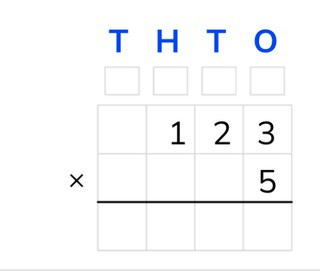Standard algorithm with a number to 1,000

# Standard algorithm with a number to 1,000

Students learn standard algorithm multiplication with a number to 1,000.

No account needed.8,000 schools use Gynzy92,000 teachers use Gynzy1,600,000 students use Gynzy

## General

The students learn standard algorithm multiplication with a number to 1,000.

## Standards

CCSS.Math.Content.5.NBT.B.5

## Learning objective

Students will be able to multiply using the standard algorithm with a number less than 10 and a number to 1,000.

## Introduction

The students quickly solve addition problems to 10,000 with simple numbers.

## Instruction

Explain that with standard algorithm multiplication you start by putting the numbers one under the other. You put the largest number on top. The T, H, T, O above the table refer to the thousands, hundreds, tens, and ones. Show that above each column there is a box for regrouping. In these boxes you can write down regrouped numbers to add them up later with the other outcomes. Explain that you start with the lower number on the right in the ones column. You multiply this number with the higher number from the ones column (5 × 3 = 15). From this 15 you write the 5 under the line in the ones column. You can write the 1 (10) in the regrouping box of the tens. Now multiply the lower number from the ones column with the higher number from the tens column (5 × 2 =10). Emphasize that you shouldn't forget to add the 1 from the regrouping box of the tens to that number (10 + 1 = 11). From this 11 you write the 1 under the line in the tens column. You can write the other 1 (100) in the regrouping box of the hundreds. Next you multiply the lower number in the ones column with the higher number from the hundreds column (5 × 1 = 5). Also, don't forget to add the 1 from the regrouping box of the hundreds to this (5 +1 = 6). Write the 6 under the line in the hundreds column. The answer is 615. Practice two problems together with the students that must be calculated using the standard algorithm. The students may choose to use a sheet of scrap paper. The students solve the next three problems on their own.

Check whether the students can multiply using the standard algorithm with a number to 1,000 by asking the following question:
- What steps do you take to solve the problem 251 × 4 using the standard algorithm?

## Quiz

The students test their understanding of standard algorithm multiplication with a number to 1,000 through ten exercises. In eight of these exercises they must solve the problems using the standard algorithm, and in the last two exercises the students must fill in the missing number.

## Closing

Discuss once again the importance of being able to multiply using the standard algorithm with a number to 1,000. As a closing activity you can have the students solve problems in pairs, in which they must calculate the second number of the problem.

## Teaching tips

Have students that have difficulty with standard algorithm multiplication with a number to 1,000 first practice standard algorithm multiplication with a number to 100. Emphasize that the students can use the regrouping boxes.

## Instruction materials

Scrap paper

### The online teaching platform for interactive whiteboards and displays in schools

• Save time building lessons

• Manage the classroom more efficiently

• Increase student engagement